# Ultimate Excel Course #1 - Excel Formulas Made Easy: Get Up to Speed with Excel Formulas Fast

#### Alan Murray, IT Trainer, Coffee Lover and Lifelong learner

Play Speed
• 0.5x
• 1x (Normal)
• 1.25x
• 1.5x
• 2x
10 Lessons (1h 11m)
• 1. Introduction

2:44
• 2. Introduction to Formulas - Writing your First Excel Formulas

7:50
• 3. The Order of Calculation - BODMAS

3:13
• 4. Formulas for Calculating Percentages

7:54
• 5. Unleash the Power of Excel Functions

8:43
• 6. Referencing other Sheets and Workbooks

11:45
• 7. Calculating Date Difference including Working Days Only

8:09
• 8. Understanding Absolute Cell Addresses

6:22
• 9. The COUNTIF Function - An Incredibly Useful Function

13:12
• 10. Thank you

0:41

## Project Description

For this class project I have multiple exercises for you to work through. Download the project-files folder to get access to the files used in the exercises.

Exercise 1 - Basic Formulas

2. Enter a formula in cell F4 to return the total in E4 minus a 10% discount. Copy the formula down to cell F17.
3. Enter a formula in cell H4 to return the number of days until the date due. Copy the formula down to cell H17.
4. Enter a SUM function in cell H1 to total the values in range F4:F17.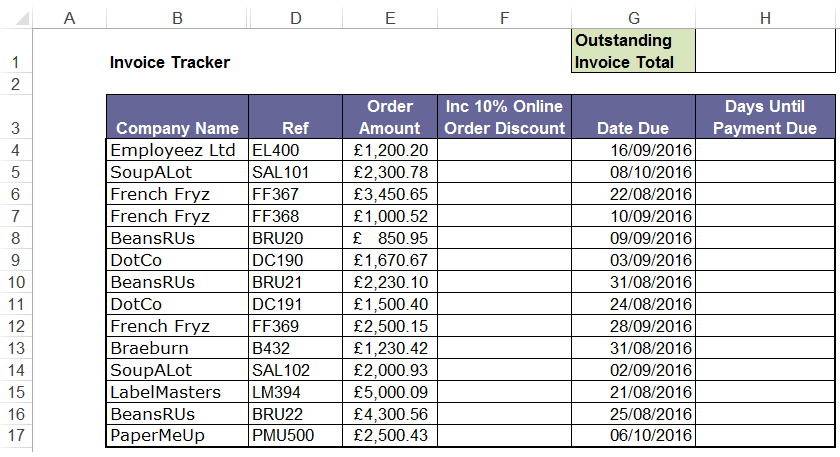Exercise 2 - Using Excel Functions

1. Open the formulas-exercise2 file.
2. Enter a function in cell H5 to return the maximum Maths exam score from the table. Copy the formula across for English, History and Geography.
3. Enter a function in cell H6 to return the minimum Maths exam score from the table and copy it across the other subjects.
4. Enter a function in cell H7 to return the average Maths exam score and copy it across.
5. Enter a COUNTIF function in cell H8 to return the number of pupils to pass Maths (pass mark is 70 or higher). Copy the formula across.
6. Enter a COUNTIF function in cell H9 to return the number of pupils who failed Maths (score is less than 70). Copy the formula across.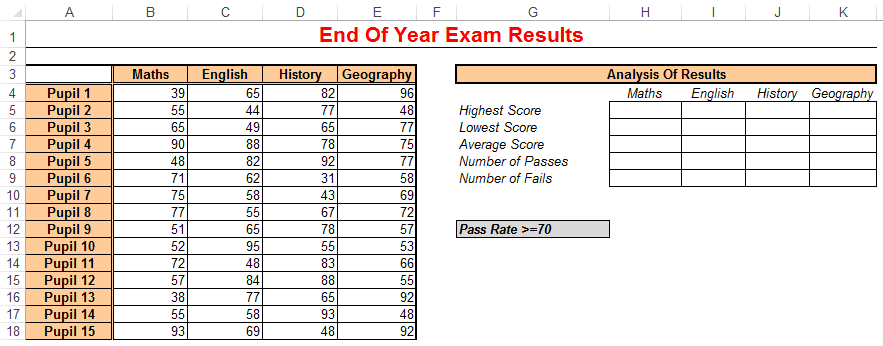Exercise 3 - Working with Absolute Cell References

1. Open the absolute-references-exercise file.
2. Enter a formula in cell D3 to calculate 20% of the total in C3. Do this by referencing the 20% in cell D1, not by typing 20% in the formula. Reference D1 in a way that when you copy the formula to cell D7 it still works correctly.
3. Enter a formula in E3 to then add the VAT in D3 to the total in C3. Copy down to E7.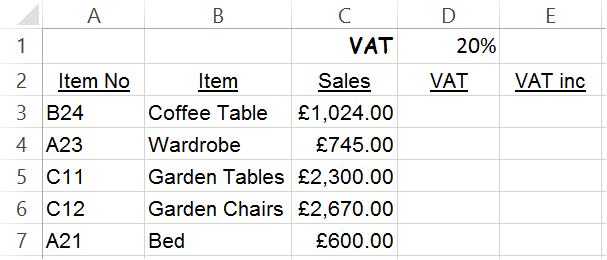1. Click on the Mixed worksheet of the absolute-references-exercise file.
2. In cell B2, Enter a formula to multiply the value in B1 by A2. Do this in a way so that if you copy the formula down to B11 and then across to column J, it continues to work correctly.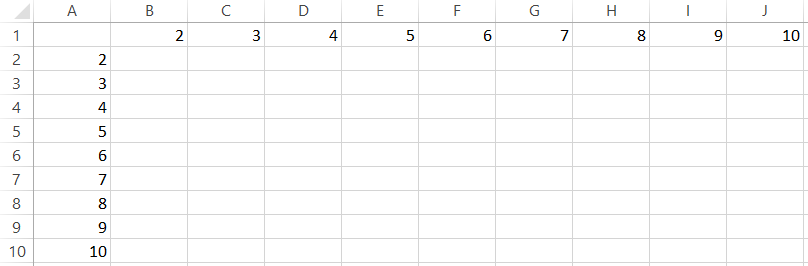Exercise 4 - Calculating percentage increase/decrease

1. Open the store-sales files.
2. In cell E6, enter a formula to calculate the percentage increase or decrease between the prior year in column C and the current year in column D. Copy the formula down to cell E20.
3. In cell G6, use the COUNTIF function to count how many stores met or exceeded the £15,000 sales target for the current year.
4. In cell G9, use the COUNTIF function to count how many stores increased sales from last year.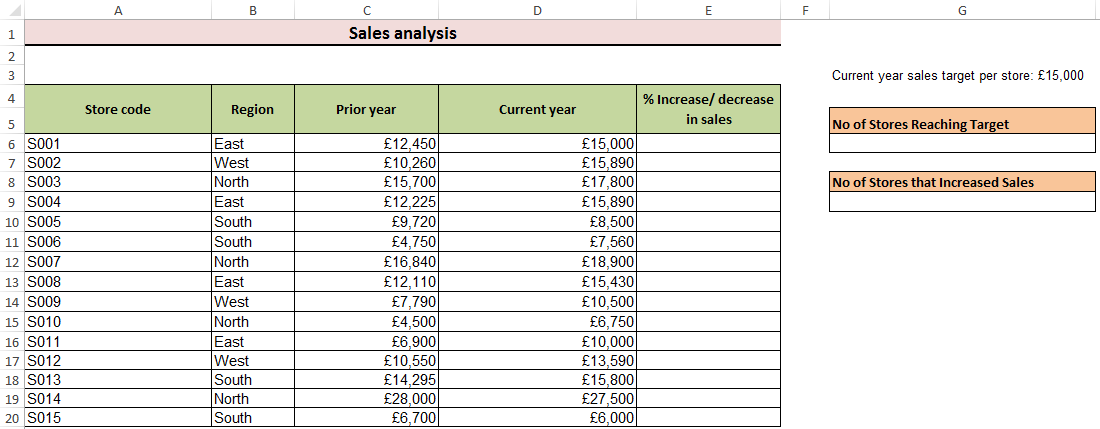75 KB